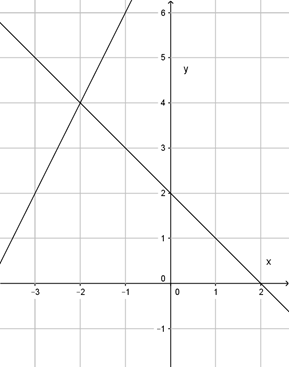• The learning process is entertaining and exciting
• The solved tasks turn into coordinates
• Along with your schoolmates and friends, go on an adventure, searching for hideouts
• E-mail:

# 2312.3. Concept of equation system

If equation system consists of two linear equations, its graphic is two lines. There can be thee substantially different arrangements of these lines on the coordinate plane. What is the solution number if these lines cross each other?

#### Look at the given graphic sketches and determine, how many solutions the system has, which is described by these equation graphics!#### Next task: 2315. Equation system concept